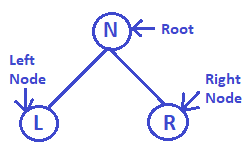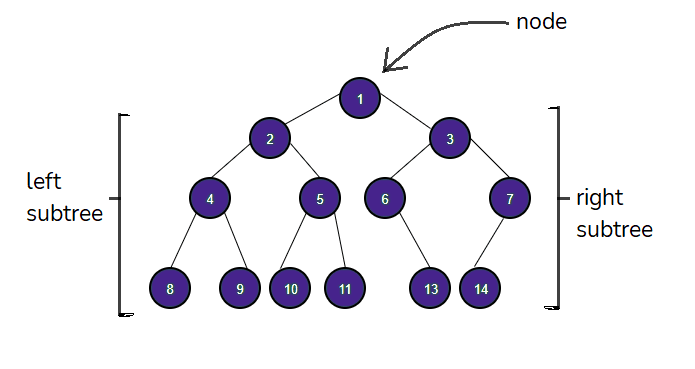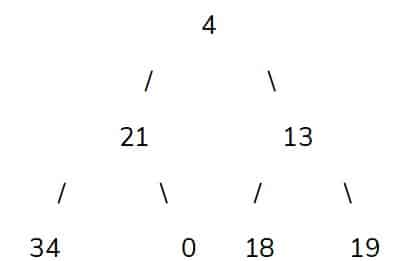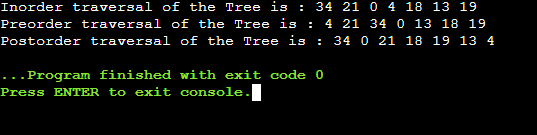# What is Tree Traversal?Do you know what are Tree Traversal Algorithms? Have you ever thought about how to implement Tree Traversal Algorithms? Let’s experience how we can use these Algorithms.

But before we jump-start our programming journey, let us know the basic meaning of the word ‘Traversal’.

The dictatorial meaning of ‘Traversal’ is to explore or to roam around. It generally means to go through to every point of a field to explore new things. Like ‘Traversing In Forest’ means to explore different points of the Forest.

The same thing goes for the programming field also. It means to go through all the nodes of that tree & perform a certain operation. The main action of this is to visit every node of the tree & execute predefined operations.

## In Which Programming Language is Tree Traversal Done?

Tree Traversal can be done in any programming language. Along with the C programming language, Tree Traversal Java is also an interesting concept. In Tree Traversal Java, the programmer needs to develop a code in Java . But the C programming language is famous for doing such operations on Tree.

But before we move ahead, let us first briefly know what is a Tree.

## What Is Tree? Read More

In the programming aspect, a Tree is a kind of Data Structure. There are two types of Data Structures present. One is a Linear type & another is a Non-Linear type. Stack and Queue are from Linear type Data Structure. Whereas, Trees are from Non-Linear Data Structures.

Mainly, a Tree is built with three components. A root (main node), left sub root (node) & right sub root (node). The root contains a certain value or information. Further left & right sub root creates a new subtree of the main tree.

## What is the Importance Of Tree Traversal?

In Linear Data Structure, traversal can be in a linear path. That is why it is quite easy to visit every point or node for that type of Data structure.

But in the case of Non-Linear Data Structures, traversing every node is not quite easy. As it is a hierarchical structure visiting every node can’t be in a linear way. This is important to perform certain operations on Trees.

Like one needs to find out the sum of the values of all nodes. Then they need to do a Tree Traversal to complete the process.

Also, it is important from another aspect. Tree Traversal Preorder can be used to sort the nodes of the Binary Tree in ascending order. Tree Traversal Preorder can apply to the Binary Trees only.

A Binary Tree is a type of Tree where the left node value will always be less than the root value. And the right node value will always be greater than the root value.

## Types Of Tree Traversal Algorithm:

There are mainly three types of Tree Traversal Algorithms. Depending upon the situation, programmers use any of them. The Tree Traversal Algorithms are:

• Tree Traversal Inorder
• Tree Traversal Preorder
• Tree Traversal Postorder

Let us know each of the methods briefly one by one.

Also, if you’re curious to know about types of constraints in linear programming then you can check out our blog section.

### Tree Traversal Inorder:

This is one type of Tree Traversal Algorithm. Here, in this case, the nodes of the tree will be visited in a particular manner. The procedure will be different from the other Algorithms.

Suppose, we have a tree where the Root is ‘N’, Left Node is ‘L’ & Right Node is ‘R’. so the Tree Traversal Inorder will visit the Root node in the middle of the two sub-nodes. The term ‘Inorder’ represents that the Root node will be inside of those two sub-nodes.

Then the Tree Traversing sequence will be:

1. Visit Left Node (L)
2. Visit Root Node (N)
3. Visit Right Node (R)

So, the remembering formula will be L-R-N. Remember this formula. This will help to solve many problems in the future.

### Tree Traversal Preorder:

This is another type of Tree Traversal Algorithm. This kind of algorithm is different from the Tree Traversal Inorder Algorithm. Let’s assume the same example.In this case, Tree Traversal Preorder will first visit the Root Node. Then it will move to the Left Sub Node. At last move to the Right Sub Node. The term ‘Preorder’ defines that the Root node will be visited first before all the preceding.

Then Tree Traversing sequence will be:

1. Visit Root Node (N)
2. Visit Left Node (L)
3. Visit Right Node (R)

So, the remembering formula will be N-L-R. Remember this formula. This will help to solve many problems in the future.

### Tree Traversal Postorder:

This is the third kind of Tree Traversing Algorithm. This is different from the previous two algorithms. Let’s take the same example for understanding purposes.In this case, Tree Traversal Postorder will first visit the Left Sub Node. Then it will directly visit the Right Sub Node. At last, it will visit the Root Node. The term ‘Postorder’ defines that the Root Node will be visited at the last of the Tree Traversal Algorithm.

Then the sequence will be:

1. Visit Left Node (L)
2. Visit Right Node (R)
3. Visit Root Node (N)

So, the remembering formula will be L-R-N. Remember this formula. This will help to solve many problems in the future.

### Implementation Of Tree Traversal Algorithm:

Implementation of the Tree Traversal Algorithm is a multistage process. It will go through different functions. Also, one needs to be very clear in function, structure, and pointer to implement such things. Also, knowing the linked list will be a cherry on the cake.

The below tree structure is going to be implemented using the code. The code will first develop the tree. Then it will do Tree Traversal Operations on it. After that, it will provide the result of Tree Traversal.The above tree will develop after providing value to it. Then the Tree Traversal Algorithms will act on it.

So, first, let us know the steps to implement such algorithms.

• Creating The Structure Of Node:

As we know, a tree is built with a value & some pointer. Here, we have taken an integer value for the info purpose. Also, we have taken two pointers of the type of the certain structure. In this way, we have created a tree structure for further use.

```// Structure For A Node Of Tree

struct node

{

// Elements Of A Node

int data;

struct node* left;

struct node* right;

};

```
• Implementing A Function to Create & Develop New Nodes:

Here, using a dynamic memory allocation process, we will create a new node. The size of the node will be equal to the size of the structure of the node. Then the data which we have collected will be inserted into the nodes. At last, the address of the node will be returned to the called function.

```/ Process To Create A New Node

struct node* createNode(int data)

{

// Allocate Memory Equivalent To Node Structure And Hold Address In Node Pointer

struct node* newNode = malloc(sizeof(struct node));

// Assigning Data To Nodes

newNode -> data = data;

newNode -> left = NULL;

newNode -> right = NULL;

// Returning The Pointer Value

return newNode;

}

```
• Linking Left & Right Sub Nodes with Root:

Here, we will define two different functions. One will add nodes to the Left side of the Root Node. Another will add nodes to the Right side of the Root Node. After that, those functions will return the address of that node to the called function.

```// Insert A New Node To Left Of The Given Node

struct node* insertLeft(struct node* root, int data)

{

root -> left = createNode(data);

return root;

}

// Insert A New Node To Right Of The Given Node

struct node* insertRight(struct node* root, int data)

{

root -> right = createNode(data);

return root;

}

```
• Adding Function for Tree Traversing Inorder Algorithm:

Here, as we previously discussed we will follow the LNR rule there. Here, printing the values of Root Node will be easy. Using the printf() function, we can print the values. But traversing to the Left & Right sub-nodes will be done using the Recursion calling of the function.

```// Process Of Inorder Traversal

void inorder(struct node* root)

{

if (root == NULL)

return;

// Recursive Calling To Follow LNR Rule

inorder(root -> left);

printf("%d ", root -> data);

inorder(root -> right);

}

```
• Adding Function for Tree Traversing Preorder Algorithm:

Here, as we previously discussed we will follow the NLR rule there. Here, printing the values of Root Node can be done using the printf() function. But traversing to the Left & Right sub-nodes will be done using the Recursion calling of the function.

```// Process Of Preorder Traversal

void preorder(struct node* root)

{

if (root == NULL)

return;

// Recursive Calling To Follow NLR Rule

printf("%d ", root -> data);

preorder(root -> left);

preorder(root -> right);

}

```
• Adding Function for Tree Traversing Postorder Algorithm:

Here, as we previously discussed we will follow the LRN rule there. Here, printing the values of Root Node can be done using the printf() function. But traversing to the Left & Right sub-nodes will be done using the Recursion calling of the function.

```// Process Of Postorder Traversal

void postorder(struct node* root)

{

if (root == NULL)

return;

// Recursive Calling To Follow LRN Rule

postorder(root -> left);

postorder(root -> right);

printf("%d ", root -> data);

}

```
• Main Function:

Here, we just need to send the values of the Root Nodes as an argument of the functions. Then we will just print the sequence of the Tree Traversal Algorithms.

```int main()

{

// Assigning Root Value

struct node* root = createNode(4);

// Assigning Other Brach Values

insertLeft(root, 21);

insertRight(root, 13);

insertLeft(root -> left, 34);

insertRight(root -> left, 0);

insertLeft(root -> right, 18);

insertRight(root -> right, 19);

// Printing The Sequence

printf("Inorder traversal of the Tree is : ");

inorder(root);

printf("\n");

printf("Preorder traversal of the Tree is : ");

preorder(root);

printf("\n");

printf("Postorder traversal of the Tree is : ");

postorder(root);

return 0;

}

```

The above is the code snippets. If needed, you can go through to the full source code there. If we run the full code, the probable output will be as follow:## Conclusion:

As we saw Tree Traversal Algorithm is very important.

We should clear the basics of structure, recursion & function calling for learning the Tree Traversal Algorithm.

Remember the short formulas of the Tree Traversal Algorithms for further problem-solving.

So, hope you have liked this piece of article. Share your thoughts in the comments section and let us know if we can improve more.

Codingzap also offers a wide range of programming and coding help services for you to benefit from. Don’t miss out! Visit https://codingzap.com/ and our expert team will be there to help you out.

If you want to know more about ‘What are Errors in C Programing’ then you can check out our article.